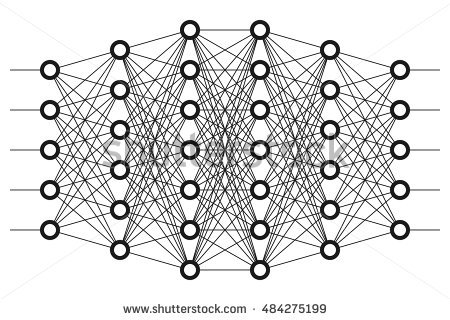# Tensorflow 1: Introduction

Here are some notes I made whilst studying Tensorflow.  I find writing out and explaining the code helps me consolidate my thoughts and refine my learning.

All my code can be found on github.  Most are presented in the form of Jupyter notebooks that i like to use.

For instructions on installing Tensorflow go here.  I installed on Ubuntu using Virtualbox and the Virtualenv method.

#### Why this guide?

There are LOTS of Tensorflow tutorials available.  I do find however that most start out basic and tend to accelerate far too quickly.  e.g. the official Tensorflow tutorial’s second lesson introduces:

• MNIST dataset
• Softmax
• Variables
• Placeholders
• Cross entropy loss function
• Batch training

Yikes!

#### First things first. What is Tensorflow?

Tensorflow is an open source library for machine learning released by Google.

It is all about creating data flow graphs.  These describe how data (tensors) move through graph nodes ( mathematical operations).

This is a two step process:

1. Build the Graph.
2. Execute the Graph.

Understanding this two step process is probably the most important early step in learning Tensorflow.  Build the Graph –> then Execute the Graph. Build the Graph –> then Execute the Graph.  Build the Graph –> then Execute the Graph.

I cannot overstate that point.

Lets build our first basic graph.

```# To use TensorFlow, we need to import the library
import tensorflow as tf```

```a = tf.constant()
b = tf.constant()```

Then lets add the two numbers together.

`c = tf.add(a,b)`

Congratulations we have a computational graph.  A very basic one to say the least.

Step 1 complete.  Now Step 2: lets execute the graph.

Remember a graph is simply defining the operations that need to be performed. Nothing has actually been computed yet.  The graph doesn’t hold any values.

To execute the graph we need to create a session.  A session is a context for executing the graph in Tensorflow.  It allocates resources and defines how and where the graph will be executed.  This might be on one computer, or multiple computers or on GPUs etc.

Lets create a session

`session = tf.Session()`

Then we tell tell the session what we want to compute.

`result = session.run(c)`

Lets print out the results

`print(result)`

Then close the session to free up the resources.

`session.close()`

Results as executed in Jupyter.  Gives the correct result of 5.Continue onto Tensors.